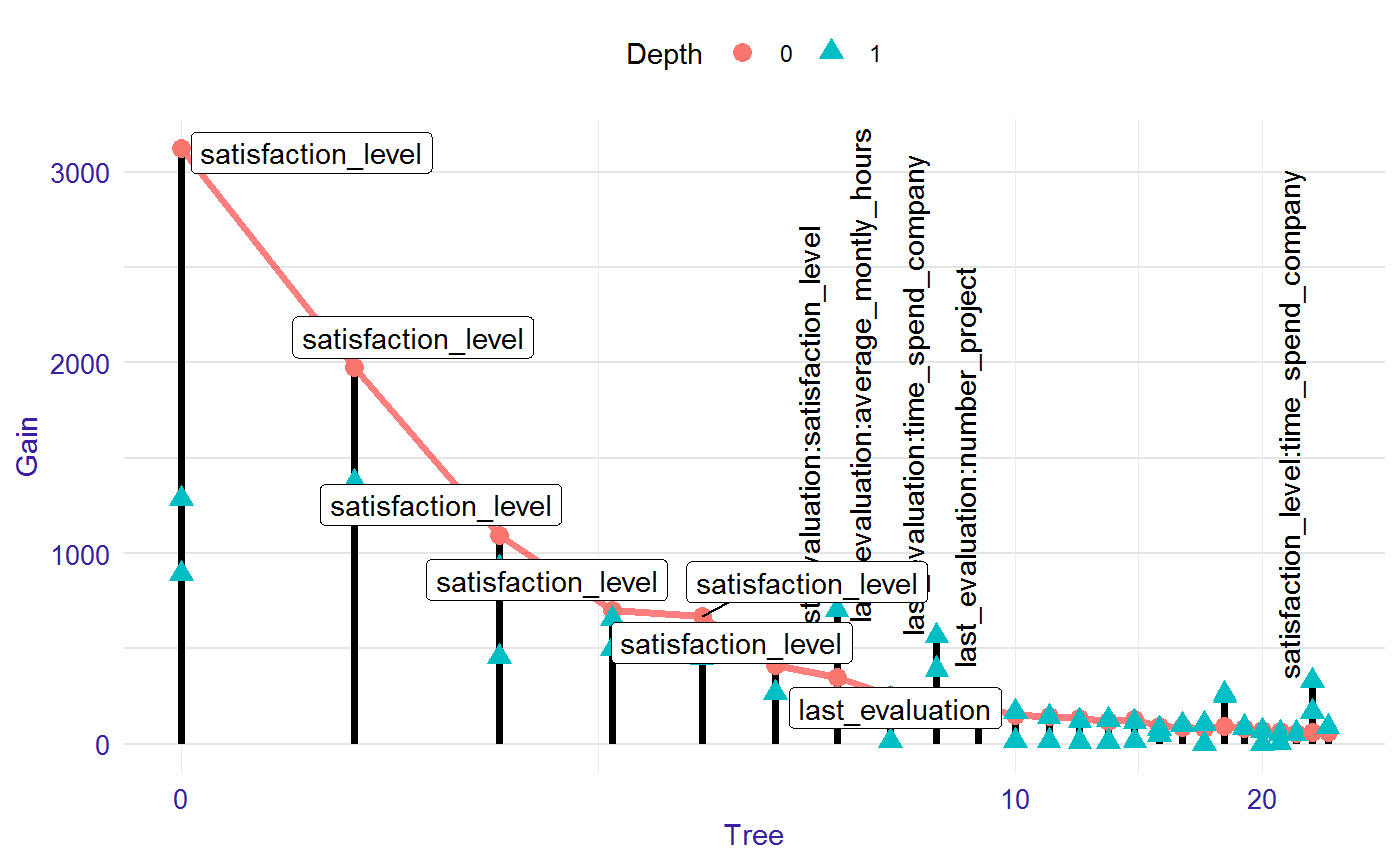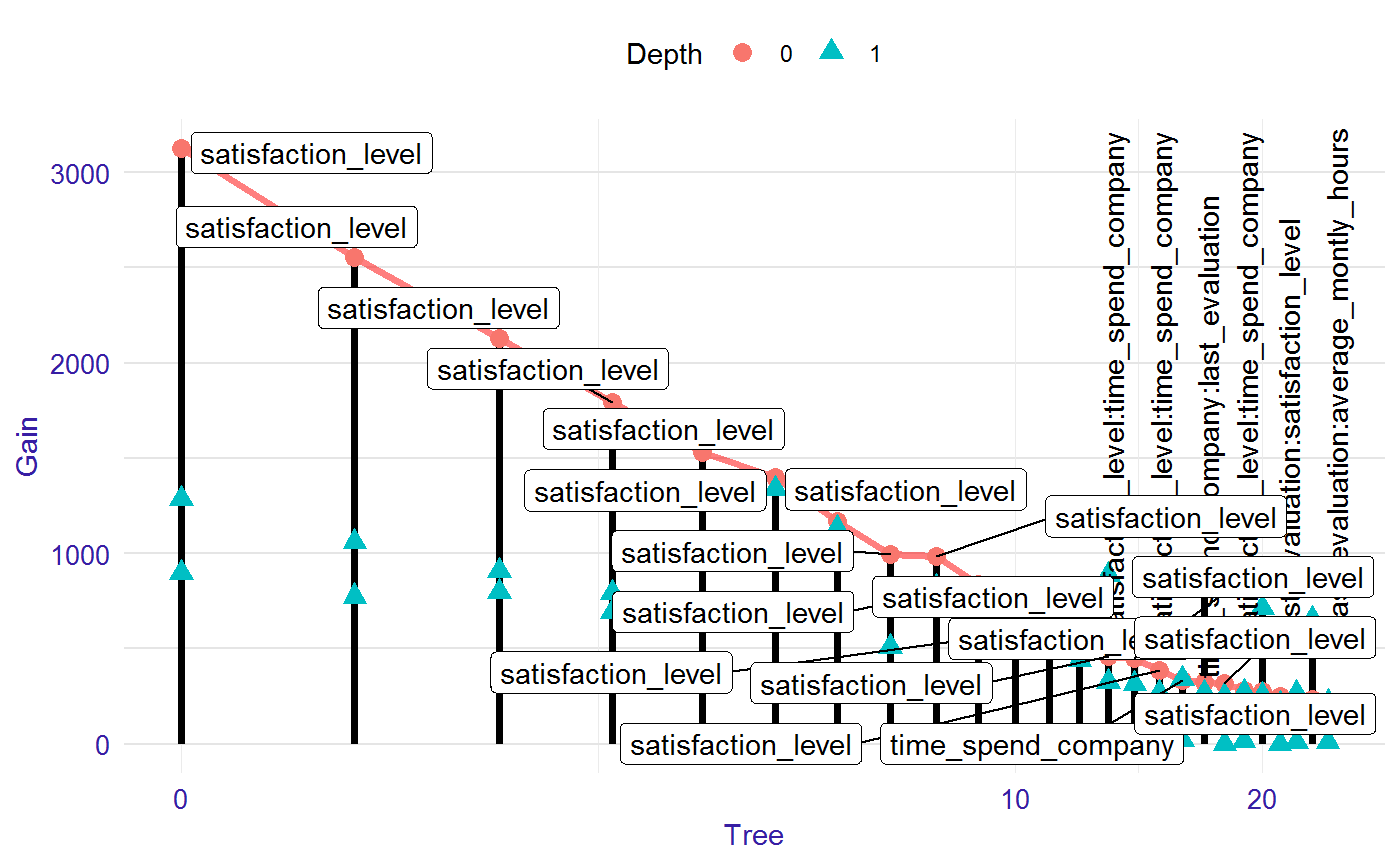This function calculates two tables needed to generate lollipop plot, which visualise the model. The first table contains information about all nodes in the trees forming a model. It includes gain value, depth and ID of each nodes. The second table contains similarly information about roots in the trees.

lollipop(xgb_model, data)

## Arguments

xgb_model a xgboost or lightgbm model. a data table with data used to train the model.

## Value

an object of the lollipop class

## Examples

library("EIX")
library("Matrix")
sm <- sparse.model.matrix(left ~ . - 1,  data = HR_data)

library("xgboost")
param <- list(objective = "binary:logistic", max_depth = 2)
xgb_model <- xgboost(sm, params = param, label = HR_data[, left] == 1, nrounds = 25, verbose = 0)

lolli <- lollipop(xgb_model, sm)
plot(lolli, labels = "topAll", log_scale = TRUE)library(lightgbm)
train_data <- lgb.Dataset(sm, label =  HR_data[, left] == 1)
params <- list(objective = "binary", max_depth = 2)
lgb_model <- lgb.train(params, train_data, 25)

lolli <- lollipop(lgb_model, sm)
plot(lolli, labels = "topAll", log_scale = TRUE)